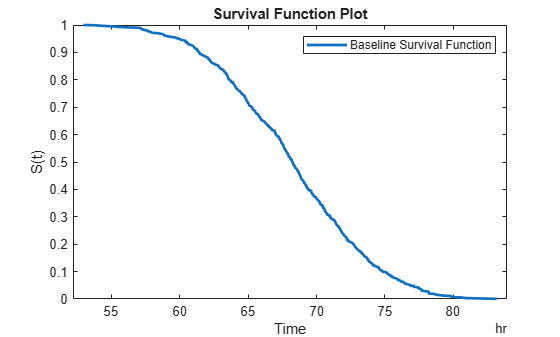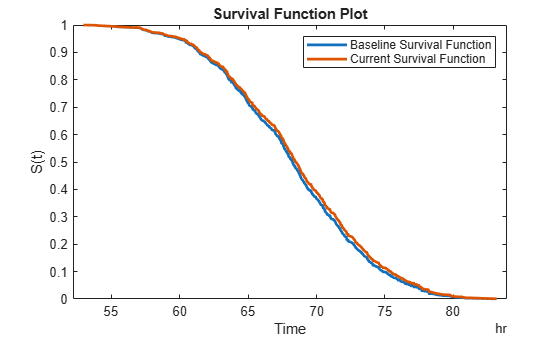# covariateSurvivalModel

Proportional hazard survival model for estimating remaining useful life

## Description

Use `covariateSurvivalModel` to estimate the remaining useful life (RUL) of a component using a proportional hazard survival model. This model describes the survival probability of a test component using historical information about the life span of components and associated covariates. Covariates are environmental or explanatory variables, such as the component manufacturer or operating conditions. Covariate survival models are useful when the only data you have is the failure times and associated covariates for an ensemble of similar components, such as multiple machines manufactured to the same specifications. For more information on the survival model, see Proportional Hazard Survival Model.

To configure a `covariateSurvivalModel` object for a specific type of component, use `fit`, which estimates model coefficients using a collection of failure-time data and associated covariates. After you configure the parameters of your covariate survival model, you can then predict the remaining useful life of similar components using `predictRUL`. For a basic example illustrating RUL prediction, see Update RUL Prediction as Data Arrives.

If you have only life span measurements and do not have covariate information, use a `reliabilitySurvivalModel`.

For general information on predicting remaining useful life, see Models for Predicting Remaining Useful Life.

## Creation

### Syntax

``mdl = covariateSurvivalModel``
``mdl = covariateSurvivalModel(initModel)``
``mdl = covariateSurvivalModel(___,Name,Value)``

### Description

example

````mdl = covariateSurvivalModel` creates a covariate survival model for estimating RUL and initializes the model with default settings.```
````mdl = covariateSurvivalModel(initModel)` creates a covariate survival model and initializes the model parameters using an existing `covariateSurvivalModel` object `initModel`.```

example

````mdl = covariateSurvivalModel(___,Name,Value)` specifies user-settable model properties using name-value pairs. For example, `covariateSurvivalModel('LifeTimeUnit',"days")` creates a covariate survival model with that uses days as a lifetime unit. You can specify multiple name-value pairs. Enclose each property name in quotes.```

### Input Arguments

expand all

Covariate survival model, specified as a `covariateSurvivalModel` object.

## Properties

expand all

Baseline hazard rate of the survival model, specified as a two-column array and estimated by the `fit` function. The second column contains the baseline survival functions values, and the first column contains the corresponding lifetime values.

For more information on the survival model, see Proportional Hazard Survival Model.

Encoding method for the categorical features in `EncodedVariables`, specified as one of the following:

• `"dummy"` — For a categorical feature with N categories, encode the variable using (N - 1) bits.

• `"binary"` — Binary encoding

You can specify `EncodingMethod`:

• Using a name-value pair when you create the model

• Using dot notation after model creation

Flag for standardizing covariate features when calculating Cox regression parameters, specified as a logical value. When `Standardize` is `true`, numeric covariate variables are standardized such that covariate `X` becomes `(X-mean(X))/std(X)`.

Standardization does not affect encoded categorical variables.

You can specify `Standardize`:

• Using a name-value pair when you create the model

• Using dot notation after model creation

Method for handling tied failure times, specified as either `"breslow"` or `"efron"`. For more information on these methods, see Cox Proportional Hazards Model.

You can specify `Ties`:

• Using a name-value pair when you create the model

• Using dot notation after model creation

Numerical and display settings for Cox regression, specified as a structure created using `statset('coxphfit')`. You can modify the options in the structure using dot notation.

You can specify `Options`:

• Using a name-value pair when you create the model

• Using dot notation after model creation

Covariate multiplying coefficients of the survival model, specified as a scalar and estimated by the `fit` function. For more information on the survival model, see Proportional Hazard Survival Model.

Covariance of the covariate multiplying coefficients, specified as a positive array with size equal to the number of coefficients and estimated by the `fit` function.

Covariate multiplying coefficient names specified as a string array and assigned when the model is trained using the `fit` function.

Coefficients corresponding to numeric covariates have the same name as the corresponding data variable in `DataVariables`. For encoded variables, the coefficient names contain the name of the corresponding encoded variable from `EncodedVariables` and a representation of the encoded bit order.

Censor variable, specified as a string that contains a valid MATLAB® variable name. The censor variable is a binary variable that indicates which life-time measurements in `data` are not end-of-life values.

`CensorVariable` must not match any of the strings in `DataVariables` or `LifeTimeVariable`.

You can specify `CensorVariable`:

• Using a name-value pair when you create the model

• As an argument when you call the `fit` function

• Using dot notation after model creation

Lifetime variable, specified as a string that contains a valid MATLAB variable name. For survival models, the lifetime variable contains the historical life span measurements of components.

You can specify `LifeTimeVariable`:

• Using a name-value pair when you create the model

• As an argument when you call the `fit` function

• Manually using dot notation

Lifetime variable units, specified as a string.

The units of the lifetime variable do not need to be time-based. The life of the test component can be measured in terms of a usage variable, such as distance traveled (miles) or fuel consumed (gallons).

Covariate data variables, specified as a string or string array. The strings in `DataVariables` must be valid MATLAB variable names. Covariates are also called environmental or explanatory variables.

You can specify `DataVariables`:

• Using a name-value pair when you create the model

• As an argument when you call the `fit` function

• Using dot notation after model creation

Encoded covariate variables, specified as a string or string array. The strings in `EncodedVariables` must be valid MATLAB variable names. Encoded variables are usually nonnumeric categorical features that `fit` converts to numeric vectors before fitting. You can also designate logical or numeric values that take values from a small set to be encoded.

To specify the method of encoding, use `EncodingMethod`.

You can specify `EncodedVariables`:

• Using a name-value pair when you create the model

• As an argument when you call the `fit` function

• Using dot notation after model creation

The strings in `EncodedVariables` must be a subset of the strings in `DataVariables`.

Additional model information for bookkeeping purposes, specified as any data type or format. The model does not use this information.

You can specify `UserData`:

• Using a name-value pair when you create the model

• Using dot notation after model creation

## Object Functions

 `predictRUL` Estimate remaining useful life for a test component `fit` Estimate parameters of remaining useful life model using historical data `plot` Plot survival function for covariate survival remaining useful life model

## Examples

collapse all

`load('covariateData.mat')`

This data contains battery discharge times and related covariate information. The covariate variables are:

• Temperature

• Manufacturer

The manufacturer information is a categorical variable that must be encoded.

Create a covariate survival model.

`mdl = covariateSurvivalModel;`

Train the survival model using the training data, specifying the life time variable, data variables, and encoded variable. There is no censor variable for this training data.

`fit(mdl,covariateData,"DischargeTime",["Temperature","Load","Manufacturer"],[],"Manufacturer")`
```Successful convergence: Norm of gradient less than OPTIONS.TolFun ```

Plot the baseline survival function for the model.

`plot(mdl)``load('covariateData.mat')`

This data contains battery discharge times and related covariate information. The covariate variables are:

• Temperature

• Manufacturer

The manufacturer information is a categorical variable that must be encoded.

Create a covariate survival model, and train it using the training data.

```mdl = covariateSurvivalModel('LifeTimeVariable',"DischargeTime",'LifeTimeUnit',"hours",... 'DataVariables',["Temperature","Load","Manufacturer"],'EncodedVariables',"Manufacturer"); fit(mdl,covariateData)```
```Successful convergence: Norm of gradient less than OPTIONS.TolFun ```

Suppose you have a battery pack manufactured by maker `B` that has run for `30` hours. Create a test data table that contains the usage time, `DischargeTime`, and the measured ambient temperature, `TestAmbientTemperature`, and current drawn, `TestBatteryLoad`.

```TestBatteryLoad = 25; TestAmbientTemperature = 60; DischargeTime = hours(30); TestData = timetable(TestAmbientTemperature,TestBatteryLoad,"B",'RowTimes',hours(30)); TestData.Properties.VariableNames = {'Temperature','Load','Manufacturer'}; TestData.Properties.DimensionNames{1} = 'DischargeTime';```

Predict the RUL for the battery.

`estRUL = predictRUL(mdl,TestData)`
```estRUL = duration 38.332 hr ```

Plot the survival function for the covariate data of the battery.

`plot(mdl,TestData)`expand all

## Version History

Introduced in R2018a